Next: 6.4 k-Space Mesh Generation Up: 6. Mesh Refinement for Previous: 6.2 Geometric Properties of

Subsections

# 6.3 Silicon Band Structure Models

Semiconductor band structures in general and especially for silicon as shown in Figure 6.4 are hard to describe with an analytical formula. The plot is drawn for energy values along particular edges of the irreducible wedge, cf. Figure 6.3(b). The energy dispersion along the straight line from point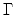to point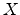, which is called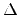-line, is marked by the red line in Figure 6.4(b).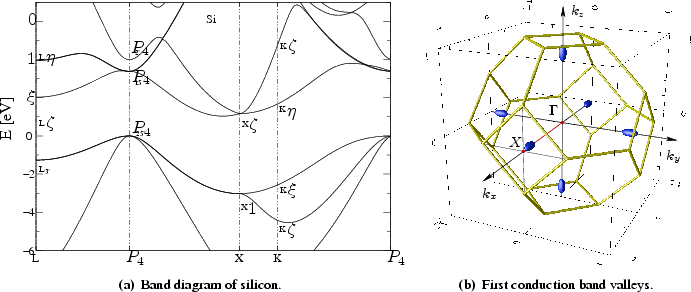For silicon the conduction band minima lie on the six equivalent-lines along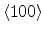-directions and occur at about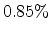of the way to the zone boundary (see Figure 6.4(b)). These are the well-known, equivalent ellipsoidal constant energy valleys. When electrons gain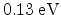of energy, they can cross the zone boundary.

There is also a second minimum in the first conduction band at point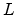, which lies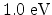about the first valley. The second conduction band valley is only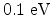above the minimum of the first conduction band. Carriers abovein kinetic energy may reside in either of the two conduction bands before the second valley of the first band is occupied. Under large electric fields, electrons populate the entire Brillouin zone and the band structure at energy minima cannot be described by simple analytical approximations.

The valence band maximum for the heavy-hole-, light-hole-, and for the split-off band is located exactly at the-point (see Figure 6.4(b)). It is obvious that for a small electric field the concentration of holes is higher in the region around the-point. For full band Monte Carlo simulations it is thus important to use a discretization of the momentum space, which shows a higher mesh density around the-point.

The complexity of semiconductor band structures forces different approximations to reduce intricacy and computational costs. Two analytical models, namely the parabolic and the non-parabolic model are widely used. The FBMC method produces a more general description, suitable also for higher energy values. This description is commonly based on the so-called pseudopotential method. These approximations, especially for valence bands, are subject of the following section.

## 6.3.1 Analytical Approximations

A brief review of known analytical band structure approximations is given to convince the reader and to make this thesis self-contained for later discussions. An all-embracing description can be found in many basic books on solid-state physics related to semiconductors such as  and .

The simplest model of the silicon band structure is based on the effective mass. If the band structure is known, the energy wave vector relation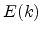in one dimension can be expanded in a Taylor series as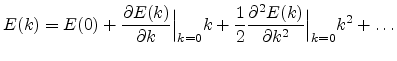(6.10)

When the band minimum occurs at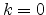, the gradient ofis zero at, so, the lowest order,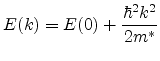(6.11)

where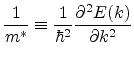(6.12)

is the effective mass, and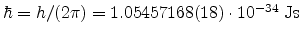is Planck's constant.

Generally for diamond crystals such as silicon (see Figure 6.1) the conduction band has three minima, one at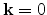(called thepoint), another along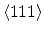directions at the boundary of the first Brillouin zone (called) and a third one near the zone boundary alongdirections. cf. Figure 6.4. If the first conduction band minimum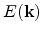is described by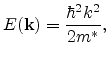(6.13)

the constant energy surface in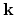-space of the band approximation, forms a sphere and the effective mass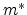is isotropic. When the conduction band does not lie at, the effective mass depends on the crystallographic orientation of the minimum. In common cubic semiconductors, we find that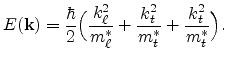(6.14)

Equation (6.14) describes a band with ellipsoidal constant energy surfaces. The effective mass is a tensor with different longitudinal and transverse effective masses,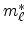and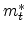, respectively. Material specific values forandcan be found e.g. in . Formula (6.14) is referred to as the parabolic energy band approximation.

In the case of high applied fields, carriers may be far above the minimum, and the higher order terms in the Taylor series expansion cannot be ignored. For the conduction band, this is approximated by a relation of the form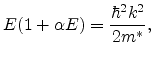(6.15)

whereis determined from formula (6.11) at the minimum. For a minimum at,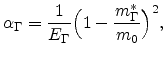(6.16)

where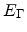is the direct bandgap. Formula (6.15) is referred to as the non-parabolic energy band approximation.

## 6.3.2 Full Band Approach

For higher energies, we can approximate the conduction band with a non parabolic parameter,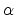, as defined in Equation (6.15). Nevertheless a non parabolic band provides a reasonable approximation only up to an energy of about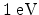. For example in silicon MOSFETs an important reliability problem is caused by injection of electrons from the channel into the gate oxide. The energy barrier at the SiO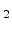: Si interface is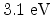. For these problems simple expressions forare not valid any more and a numerically generated table ofmust be used.

A very useful approach to a numerical evaluation ofis the so called pseudopotential method . To evaluatethe Schrödinger equation for the electrons is solved for a bulk semiconductor in the absence of scattering and without any built-in potential . The pseudopotential method relies on the fact that the band structure is largely determined by the valence electrons. In addition empirical form factors have been derived to fit band gaps at the high symmetry locations. Using this empirical pseudopotential method, the energy band structures of most common semiconductors have been evaluated and they are available in tabular form.

Remark 5 (Full Band Approach)   In the following the numerical evaluation ofby the pseudopotential method and the storage of energy band values related to the tessellation of the first Brillouin zone in-space is referred simply as full band approach. Other meanings or specifications are pointed out explicitely.Next: 6.4 k-Space Mesh Generation Up: 6. Mesh Refinement for Previous: 6.2 Geometric Properties of

Wilfried Wessner: Mesh Refinement Techniques for TCAD Tools